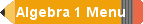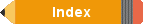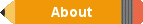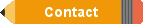Algebra 1 Chapter 00 Review of prior years of Math
 Pre Ch 0 Chapter 0 Preparing for Algebra 1 0.1 Plan for Problem Solving 0.2 Real Numbers 0.3 Operations with Integers 0.4 Adding and Subtracting Rational Numbers 0.5 Multiplying and Dividing Rational 0.6 The Percent Proportion 0.7 Perimeter 0.8 Area 0.9 Volume 0.10 Surface Area 0.11 Simple Probability and Odds Post Ch 0 Test
Chapter 0 in Algebra 1 is essentially a review of
math consisting of parts from Math 6, Math 7 and
Math 8 which are typically taken in middle school
grades 6, 7 and 8 respectively. Student's are
expected to know this material prior to taking
Algebra 1; otherwise taking Algebra 1 may be too
difficult.